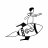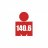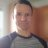# Create Watchlist Column for Stochastic

H

##### New member
There is a useful column study shared on here: https://usethinkscript.com/threads/...ossover-watchlist-column-for-thinkorswim.191/

I wanted to use this for Stochastic instead because I only want to look for long trades in my list of stocks when K crosses over D to the upside. K is the thinner line, D is the thicker line. I need the look back period to be zero (which doesn't seem to work), as I want to know if the stochastic is -currently- red and angling down, or -currently- green and angling up.

Summarily, long when D is green, short when D is red.I am hoping that one of the experts in here could adjust the code in a way that makes it work. I know this is asking a lot as a stranger, but I have made several attempts at getting the result myself and only fall short each time.

FWIW here is my most recent attempt. It's embarrassing, I know:

Code:
``````declare lower;

input lookback= 1;
input over_bought = 80;
input over_sold = 20;
input KPeriod = 5;
input DPeriod = 3;
input priceH = high;
input priceL = low;
input priceC = close;
input slowing_period = 2;
input averageType = AverageType.SIMPLE;
input showBreakoutSignals = {default "No", "On FullK", "On FullD", "On FullK & FullD"};

def lowest_k = Lowest(priceL, KPeriod);
def c1 = priceC - lowest_k;
def c2 = Highest(priceH, KPeriod) - lowest_k;
def FastK = if c2 != 0 then c1 / c2 * 100 else 0;

def FullK = MovingAverage(averageType, FastK, slowing_period);
def FullD = MovingAverage(averageType, FullK, DPeriod);

def bull_cross = FullK crosses above FullD;
def bear_cross = FullK crosses below FullD;

def bull_lookback = highest(bull_cross, lookback);
def bear_lookback = highest(bear_cross, lookback);

plot signal = if bull_lookback then 2 else if bear_lookback then 1 else 0;
signal.AssignValueColor(if signal == 2 then Color.Dark_Green

else if signal == 1 then Color.Dark_Red else Color.Dark_Orange);
AssignBackgroundCOlor(if signal == 2 then Color.Dark_Green

else if signal == 1 then Color.Dark_Red else Color.Dark_Orange);``````

Last edited:#### hectorgasm

##### New member
Found this code on the web, i hope you find it useful. Also watch on the upper right side the FX Price Type, make sure it is the same as the one you have in your chart.

Code:
``````#######

input over_bought = 80;
input over_sold = 20;
input KPeriod = 10;
input DPeriod = 10;
input priceH = high;
input priceL = low;
input priceC = close;
input slowing_period = 3;
input averageType = AverageType.SIMPLE;
def lowest_k = Lowest(priceL, KPeriod);
def c1 = priceC – lowest_k;
def c2 = Highest(priceH, KPeriod) – lowest_k;
def FastK = if c2 != 0 then c1 / c2 * 100 else 0;
def FullK = MovingAverage(averageType, FastK, slowing_period);
def FullD = MovingAverage(averageType, FullK, DPeriod);
#plot OverBought = over_bought;
#plot OverSold = over_sold;
#FullK.SetDefaultColor(GetColor(5));
#FullD.SetDefaultColor(GetColor(0));
#OverBought.SetDefaultColor(GetColor(1));
#OverSold.SetDefaultColor(GetColor(1));
#AssignPriceColor (if Fullk > FullD then color.green else color.red);
AssignBackgroundColor(if Fullk > FullD then color.green else color.red);
plot value = FullK;``````

•mrmac
H

##### New member
Thanks a lot @hectorgasm this is great. I don't know what you mean by "upper right side the FX Price Type?" I'm only trading equities so I think it's fine as is as far as I can see right now -#### MBF

##### Active member
2019 Donor
Sigh .... hey @BenTen, I am working on this indicator for my watchlist. This is what I have but it is not correct. IF you fix it for me could you explain where I went wrong, please? I am trying to get a red color box when the 1 hour (include after hours) stochslow (k=5) crosses below 81. SOS.
#StochasticSlow("k period" = 5, "d period" = 1)."SlowK" crosses below 81
Input StochasticSlow = 5;
Input stochasticCrossover = 81;

def StochasticSlowvalue = close  < stochasticslow and close > stochasticslow;
def stochasticCrossovervalue = close  < stochasticCrossovervalue  and close > stochasticCrossover;

plot scan = stochasticCrossovervalue;

assignBackgroundColor (if scan <= 1 then color.GREEN else color.BLACK);
scan.assignvaluecolor(if stochasticcrossovervalue >= 1 then color.BLACK else color.GREEN);##### Well-known member
VIP
@MBF this code was created using the Conditional Wizard and Script Editor...

Ruby:
``````plot xover = StochasticSlow("k period" = 5, "d period" = 1)."SlowK" crosses below 80;
xover.AssignValueColor(if xover == 1 then color.green else color.black);
AssignBackgroundColor(if xover == 1 then color.green else color.black);``````

••BenTen and MBF#### MBF

##### Active member
2019 Donor
I sure know how to complicate things. Thank you @rad14733Is it possible to create a scanner for new daily highs? Questions 0Create Strategy to open position on 3rd candle above 9 SMA Questions 6Need to create an audible alert for new 10-minute HIGH/LOW... Questions 5